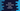# zip and unzip methods in Kotlin array## Introduction :

zip function builds one new list of paired elements from two existing arrays. Zipping transformation is useful to combine two array values. The final result is equal to the length of the smaller array if both arrays have different size. The extra elements of the larger array are not included in the final list.

Similarly, to do the reverse transformation, i.e. unzipping, unzip() method is called. In this post, I will show you how to use zip and unzip in Kotlin with examples.

### zip :

zip function is defined as below :

``fun <T,R> Array<t>.zip(second: Array<r>) : List<Pair<T,R>>``

It returns one list of pairs built from the caller and the second array. It uses the same index from both arrays and the final list length is equal to the length of the shorter array.

### Example :

``````fun main() {
val firstArray = intArrayOf(1, 2, 3, 4, 5)
val secondArray = arrayOf("one", "two", "three", "four", "five")
val thirdArray = arrayOf('a', 'b', 'c', 'd', 'e', 'f', 'g', 'h', 'i', 'j')

println(firstArray zip secondArray)
println(secondArray zip thirdArray)
}``````

### Output :

``````[(1, one), (2, two), (3, three), (4, four), (5, five)]
[(one, a), (two, b), (three, c), (four, d), (five, e)]``````

Here, firstArray and secondArray has five elements and thirdArray has ten elements. Zipping firstArray and secondArray results one list of five elements and zipping secondArray and thirdArray results one list of five elements. The last five elements of thirdArray are ignored.

### zip with transform :

zip method has one more variant, that takes one transform function as the second argument :

``fun <T,R> Array<t>.zip(second: Array<r>, transform: (a:T, b:R) -> R) : List<Pair<T,R>>``

The transformation function takes two parameters. This function is called on each pairs of the list and result list contains values generated by this transform function.

``````fun main() {
val firstArray = intArrayOf(1, 2, 3, 4, 5)
val secondArray = arrayOf("one", "two", "three", "four", "five")
val thirdArray = arrayOf('a', 'b', 'c', 'd', 'e', 'f', 'g', 'h', 'i', 'j')

println(firstArray.zip(secondArray) { a, b -> "\$a-\$b" })
println(secondArray.zip(thirdArray) { a, b -> "\$a:\$b" })
}``````

It will print :

``````[1-one, 2-two, 3-three, 4-four, 5-five]
[one:a, two:b, three:c, four:d, five:e]``````

### Unzipping zipped values :

The reverse operation of zip is unzip(). This method provides two lists from this pairs. The first list contains the first elements of each pairs in the list and the second list contains the second elements of each pairs in the list.

#### For example :

``````fun main() {
val zippedList = listOf(1 to "one", 2 to "two", 3 to "three", 4 to "four", 5 to "five")
val unzippedList = zippedList.unzip()

println(unzippedList.first)
println(unzippedList.second)
}``````

It will print :

``````[1, 2, 3, 4, 5]
[one, two, three, four, five]``````

### All examples :

``````fun main() {
val firstArray = intArrayOf(1, 2, 3, 4, 5)
val secondArray = arrayOf("one", "two", "three", "four", "five")
val thirdArray = arrayOf('a', 'b', 'c', 'd', 'e', 'f', 'g', 'h', 'i', 'j')

println(firstArray zip secondArray)
println(secondArray zip thirdArray)

println(firstArray.zip(secondArray) { a, b -> "\$a-\$b" })
println(secondArray.zip(thirdArray) { a, b -> "\$a:\$b" })

val zippedList = listOf(1 to "one", 2 to "two", 3 to "three", 4 to "four", 5 to "five")
val unzippedList = zippedList.unzip()

println(unzippedList.first)
println(unzippedList.second)
}``````

#### Output :

``````[(1, one), (2, two), (3, three), (4, four), (5, five)]
[(one, a), (two, b), (three, c), (four, d), (five, e)]
[1-one, 2-two, 3-three, 4-four, 5-five]
[one:a, two:b, three:c, four:d, five:e]
[1, 2, 3, 4, 5]
[one, two, three, four, five]``````